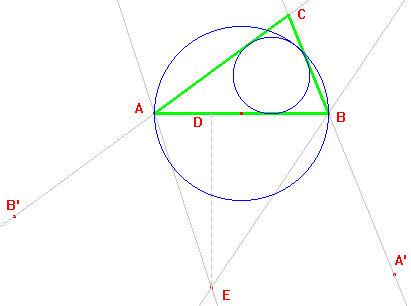c15

Triangles ABC given A and B and such as the circle with diameter AB is tangent to the incircle

1) Take D in AB.
2) Take E in the perpendicular from D to AB, such as ED = AB.
3) Let B' be the reflection of B on AE.
4) Let A' be the reflection of A on BE.
5) The lines AB' and A'B intersect at C; ABC is the requested triangle.